To Top

## 1. cnn-challenges

CNN善于检测特征，却在探索特征（视角，大小，方位）之间空间关系方面效果较差。(一个简单的CNN模型可以正确提取鼻子、眼睛和嘴巴的特征，但如果一张图里，鼻子和眼睛错位了，或者一只眼睛倾斜了一定的角度，那这张图仍然有可能错误地激活神经元导致认为这张图就是人脸)

## 2. equivariance

• Invariance对应特征检测，特征是不变的。例如，检测鼻子的神经元不管什么方向，都检测鼻子。但是，神经元空间定向的损失最终会损害这种invariance模型的有效性。
• Equivariance对应变体检测，也即可以相互转换的对象（例如检测不同方向的人脸）。直观地说，胶囊网络检测到脸部旋转了20°，而不是实现与旋转了20°的变体相匹配的脸。通过强制模型学习胶囊中的特征变体，我们可以用较少的训练数据更有效地推断可能的变体。此外，也可以更有效地防止对抗攻击

## 3. capsule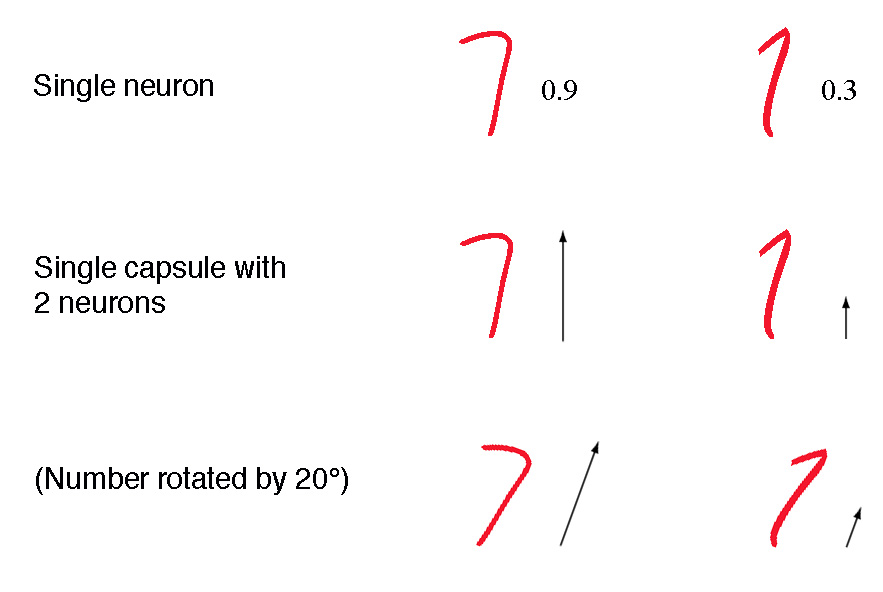## 4. dynamic-routing

### 4.2 calculating-a-capsule-output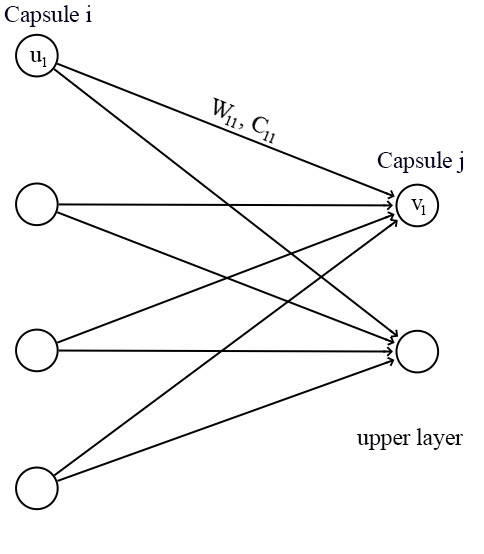$\\ \hat u_{j|i}=W_{ij}u_i \\ s_j=\sum_i c_{ij}\hat u_{j|i}$

$v_j=\frac{||s_j||^2}{1+||s_j||^2}\frac{s_j}{||s_j||}$

$\\ v_j\approx ||s_j||s_j,\ for\ s_j\ is\ short \\ v_j\approx \frac{s_j}{||s_j||},\ for\ s_j\ is\ long$

## 5. iterative-dynamic-routing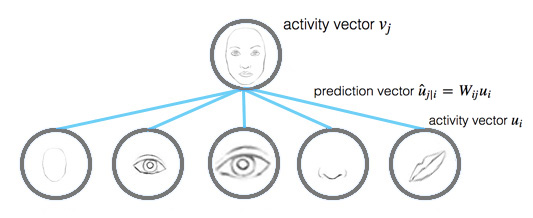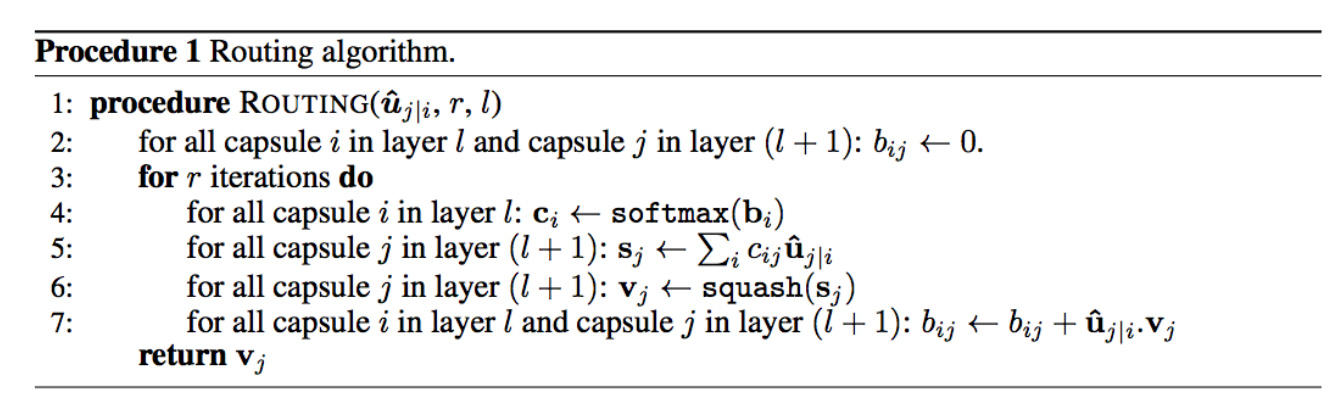## 8. capsnet-architecture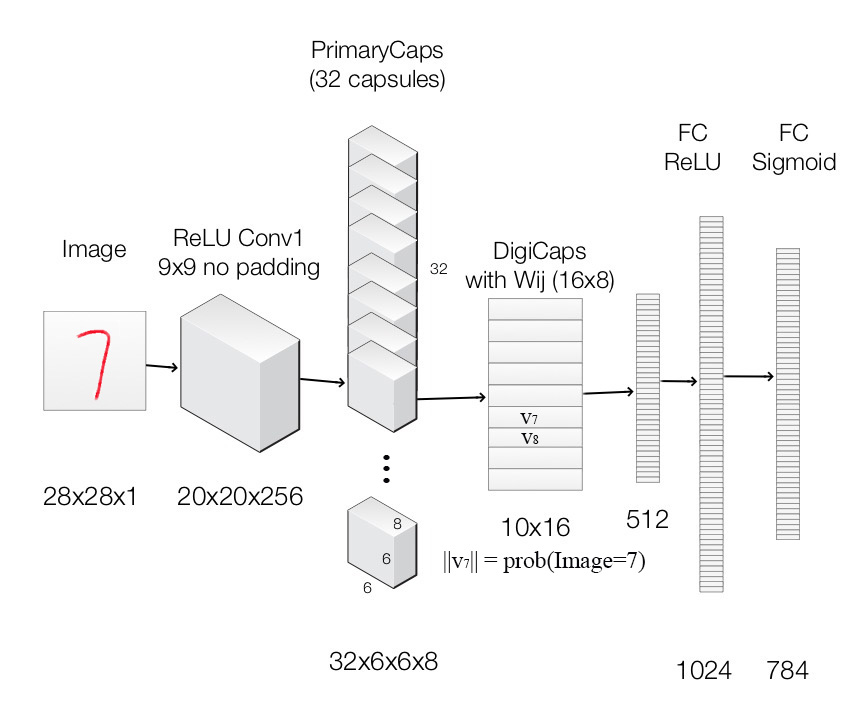Layer Name Apply Output shape
Image Raw image array 28x28x1
ReLU Conv1 Convolution layer with 9x9 kernels output 256 channels, stride 1, no padding with ReLU 20x20x256
PrimaryCapsules Convolution capsule layer with 9x9 kernel output 32x6x6 8-D capsule, stride 2, no padding 6x6x32x8
DigiCaps Capsule output computed from a (16x8 matrix) between and ( from 1 to 32x6x6 and from 1 to 10). 10x16
FC1 Fully connected with ReLU 512
FC2 Fully connected with ReLU 1024
Output image Fully connected with sigmoid 784(28x28)

## 12. sarasra的代码

python layers_test.py


### 12.1 Quick mnist test results：

• 下载tfrecords，并解压到$DATA_DIR/ wget https://storage.googleapis.com/capsule_toronto/mnist_data.tar.gz  • 下载model checkpoint，并解压到$CKPT_DIR
wget https://storage.googleapis.com/capsule_toronto/mnist_checkpoints.tar.gz

• 测试
python experiment.py --data_dir=$DATA_DIR/mnist_data/ --train=false \ --summary_dir=/tmp/ --checkpoint=$CKPT_DIR/mnist_checkpoint/model.ckpt-1


### 12.2 Quick CIFAR10 ensemble test results

• 下载cifar10 binary version，并解压到$DATA_DIR/ wget https://www.cs.toronto.edu/~kriz/cifar.html  • 下载cifar10 model checkpoints，并解压到$CKPT_DIR
wget https://storage.googleapis.com/capsule_toronto/cifar_checkpoints.tar.gz

• 测试
python experiment.py --data_dir=$DATA_DIR --train=false --dataset=cifar10 \ --hparams_override=num_prime_capsules=64,padding=SAME,leaky=true,remake=false \ --summary_dir=/tmp/ --checkpoint=$CKPT_DIR/cifar/cifar{}/model.ckpt-600000 \
--num_trials=7


### 12.3 训练

• mnist
python experiment.py --data_dir=$DATA_DIR --dataset=cifar10 --max_steps=600000\ --hparams_override=num_prime_capsules=64,padding=SAME,leaky=true,remake=false \ --summary_dir=/tmp/  • mnist baseline python experiment.py --data_dir=$DATA_DIR/mnist_data/ --max_steps=300000\
--summary_dir=/tmp/attempt1/ --model=baseline

• cifar
python experiment.py --ata_dir=$DATA_DIR/mnist_data/ --max_steps=300000\ --summary_dir=/tmp/attempt0/  ### 12.4 训练+验证 训练时在验证集上验证： • --validate=true • 需要两个gpu：一个训练；一个验证 • 如果两个job都在同一台机器，需要限制每个job的RAM大小，因为 TensorFlow will fill all your RAM for the session of your first job and your second job will fail python experiment.py --data_dir=$DATA_DIR/mnist_data/ --max_steps=300000\
--summary_dir=/tmp/attempt0/ --train=false --validate=true


--num_targets=2
--data_dir=$DATA_DIR/multitest_6shifted_mnist.tfrecords@10  生成multiMNIST/MNIST records的代码： input_data/mnist/mnist_shift.py  generate multiMNIST test split的代码： python mnist_shift.py --data_dir=$DATA_DIR/mnist_data/ --split=test --shift=6


build expanded_mnist for affNIST generalizability：

--shift=6 --pad=6


comment here..